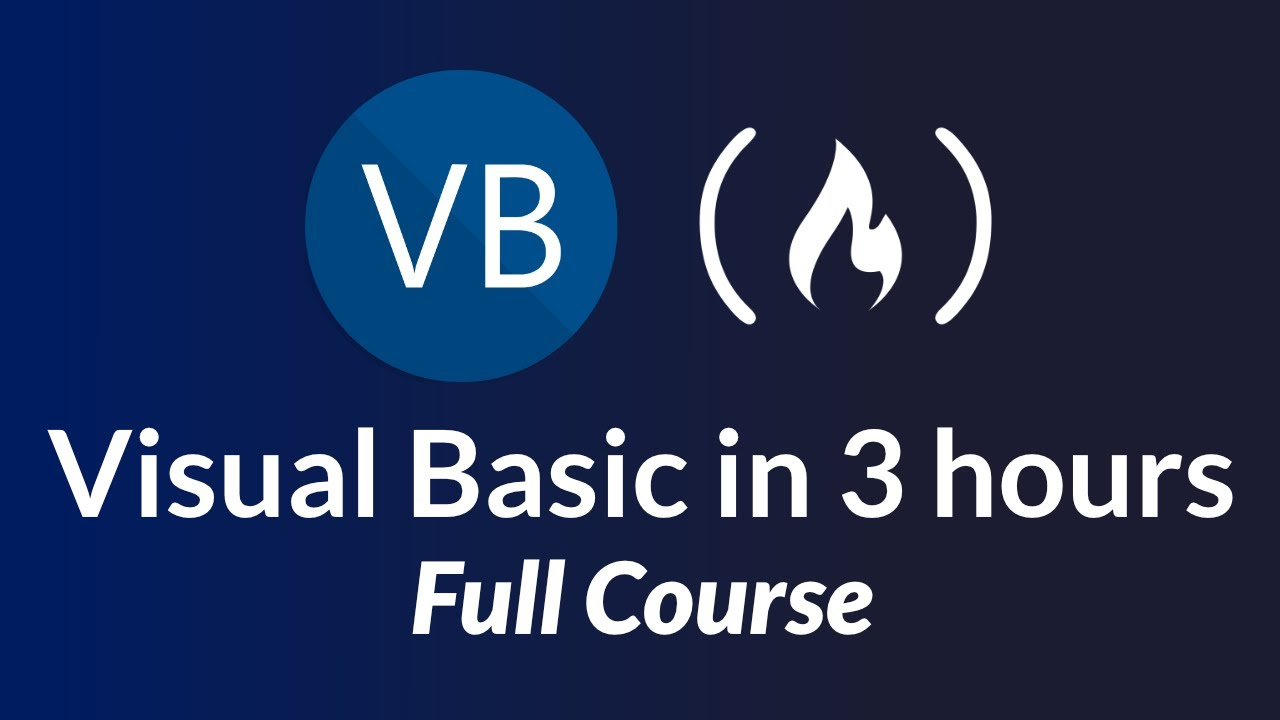# Visual Basic (VB.NET) – Full Course for BeginnersLean the fundamentals of programming with Visual Basic (sometimes called Visual Basic .NET or VB.NET).

Lean the fundamentals of programming with Visual Basic (sometimes called Visual Basic .NET or VB.NET).

In this tutorial, you will learn about the basic constructs of high level programming languages, including sequence, selection and iteration. You will learn how to build an event-driven, form-based, user interface to capture input, and you will learn how to write code to validate and process the data collected.

⭐️ Course Contents ⭐️
⌨️ (0:00:00) Introduction
⌨️ (0:00:46) Hello Visual Studio
⌨️ (0:13:44) Customise The Visual Studio IDE
⌨️ (0:19:13) Output and Variables
⌨️ (0:34:26) Variable Data Types
⌨️ (0:41:56) Input with Windows Forms
⌨️ (0:54:18) Debugging Code
⌨️ (1:02:47) Arithmetic Operators
⌨️ (1:14:24) Complex Arithmetic Expressions
⌨️ (1:22:50) Selection with If Statements
⌨️ (1:32:58) Logical and Relational Operators 1
⌨️ (1:47:32) Logical and Relational Operators 2
⌨️ (1:56:37) Select Case
⌨️ (2:02:57) For Next
⌨️ (2:08:17) Practice For Next Loops & If Blocks
⌨️ (2:12:39) Do While
⌨️ (2:21:24) Condition Controlled Loops
⌨️ (2:29:39) Array Variables
⌨️ (2:39:48) Practice Arrays & Loops
⌨️ (2:49:46) Linear Search
⌨️ (2:56:24) Two Dimensional Arrays
⌨️ (3:07:00) 2D Arrays & Nested Loops